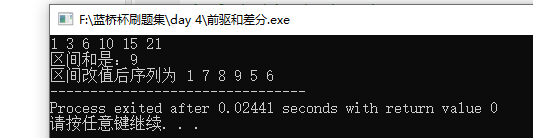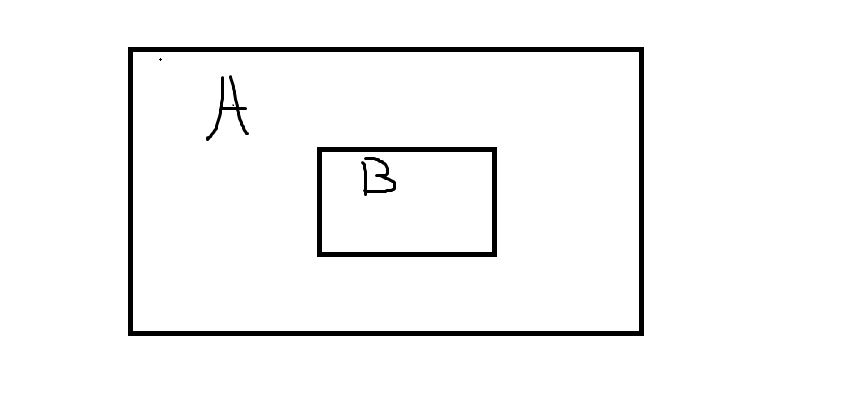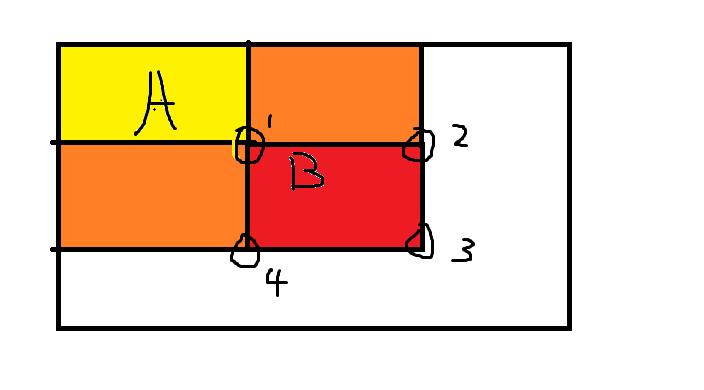# 蓝桥杯刷题之分享方法---前缀差分# 一、一维数组的前缀与差分

	我用一道题来解释吧题目如下：
有一个1到6的序列   等差
计算2到第四个数的和
将2 到第四个数每个数都+5


#include<bits/stdc++.h>
using namespace std;
int s,d;
int a={0,1,2,3,4,5,6};
int main(){
for(int i=1;i<=6;i++){
s[i]=s[i-1]+a[i];
d[i]=a[i]-a[i-1];
cout<<s[i]<<" ";//计算前驱
}
cout<<endl;
int res = 0;
res=s-s[2-1];
cout<<"区间和是："<<res<<endl;
//差分
d+=5; //让首项和前一项的差加五
d[4+1] -= 5;//就是让第五项与第四项的差减五
cout<<"区间改值后序列为 ";
//一步好好理解哦
for(int i =1;i<=6;i++){
s[i] = s[i-1]+d[i];
cout<<s[i]<<" ";
}

return 0;

}# 二、激光炸弹

地图上有 N 个目标，用整数 Xi,Yi 表示目标在地图上的位置，每个目标都有一个价值 Wi。



### 输出格式

#include<bits/stdc++.h>
using namespace std;

const int N=5050;
int s[N][N];
int main()
{
int N,R;
cin>>N>>R;
int n=R,m=R;
for(int i=0;i<N;i++)
{
int x,y,w;
cin>>x>>y>>w;
x++,y++;
s[x][y]+=w;
n=max(n,x),m=max(m,y);//至少是R*R初坐标
}
//求二维前缀和
for(int i=1;i<=n;i++)
for(int j=1;j<=m;j++)
s[i][j]=s[i-1][j]+s[i][j-1]-s[i-1][j-1]+s[i][j];

//求边长为R的正方形的最大值
int res=0;
for(int i=R;i<=n;i++)
for(int j=R;j<=m;j++)
res=max(res,s[i][j]-s[i-R][j]-s[i][j-R]+s[i-R][j-R]);
//这一步相信大家了解了 二维的前缀和计算方法就不难了
cout<<res<<endl;
return 0;
}s[i][j]=s[i-1][j]+s[i][j-1]-s[i-1][j-1]+s[i][j];


# 关于小编

03-253万+08-033万+
02-2174
03-319185
03-161044
02-253499
08-12427
09-22361
04-2288
03-1817
08-172万+
03-0351
10-131万+
04-192246
12-052686
04-0318
10-29178
02-05124
04-181911
©️2020 CSDN 皮肤主题: 1024 设计师:白松林